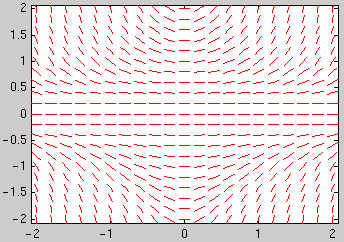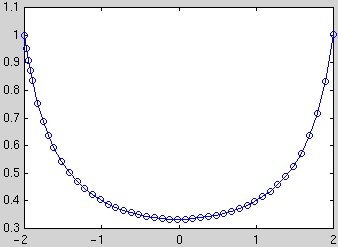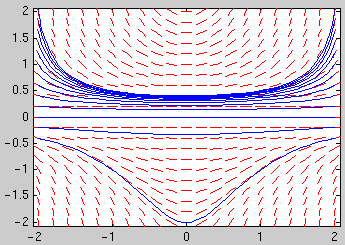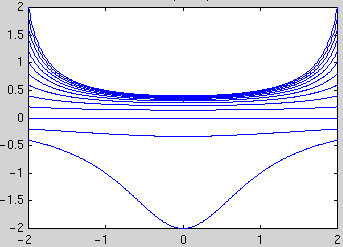# Using Matlab for First Order ODEs

## Contents

Inline functions
Direction fields
Numerical solution of initial value problems
Plotting the solution
Combining direction field and solution curves
Finding numerical values at given t values
Symbolic solution of ODEs
Finding the general solution
Solving initial value problems
Plotting the solution
Finding numerical values at given t values

## Inline Functions

If you want to use a function several times it is convenient to define it as a so-called inline function:

`f1 = inline('sin(x)*x','x')`

defines the function `f1(x)=sin(x)*x`. Note that the arguments of `inline` must be strings (not symbolic expressions). You can then use the function `f1` in expressions you type in.

You can also define inline functions of several variables:

`g1 = inline('x*y+sin(x)','x','y')`

defines the function `g1(x,y)=x*y+sin(x)` of two variables.

## Direction Fields

First download the file `dirfield.m` and put it in the same directory as your other m-files for the homework.

Define an inline function `g` of two variables `t`, `y` corresponding to the right hand side of the differential equation y'(t) = g(t,y(t)). E.g., for the differential equation y'(t) = t y2 define

`g = inline('t*y^2','t','y')`

You have to use `inline(...,'t','y')`, even if `t` or `y` does not occur in your formula.

To plot the direction field for t going from t0 to t1 with a spacing of dt and y going from y0 to y1 with a spacing of dy use `dirfield(g,t0:dt:t1,y0:dy:y1)`. E.g., for `t` and `y` between -2 and 2 with a spacing of 0.2 type

`dirfield(g,-2:0.2:2,-2:0.2:2)`## Solving an initial value problem numerically

First define the inline function `g` corresponding to the right hand side of the differential equation y'(t) = g(t,y(t)). E.g., for the differential equation y'(t) = t y2 define

`g = inline('t*y^2','t','y')`

To plot the numerical solution of an initial value problem: For the initial condition y(t0)=y0 you can plot the solution for t going from t0 to t1 using `ode45(g,[t0,t1],y0)`.

Example: To plot the solution of the initial value problem y'(t) = t y2, y(-2)=1 in the interval [-2,2] use

`ode45(g,[-2,2],1)`The circles mark the values which were actually computed (the points are chosen by Matlab to optimize accuracy and efficiency). You can obtain vectors `ts` and `ys` with the coordinates of these points using `[ts,ys] = ode45(g,[t0,t1],y0)`. You can then plot the solution using `plot(ts,ys)` (this is a way to obtain a plot without the circles).

To combine plots of the direction field and several solution curves use the commands ```hold on``` and `hold off`: After obtaining the first plot type `hold on`, then all subsequent commands plot in the same window. After the last plot command type `hold off`.

Example: Plot the direction field and the 13 solution curves with the initial conditions y(-2) = -0.4, -0.2, ..., 1.8, 2:

```dirfield(g,-2:0.2:2,-2:0.2:2)
hold on
for y0=-0.4:0.2:2
[ts,ys] = ode45(g,[-2,2],y0); plot(ts,ys)
end
hold off```To obtain numerical values of the solution at certain t values: You can specify a vector `tv` of t values and use `[ts,ys] = ode45(g,tv,y0)`. The first element of the vector `tv` is the initial t value; the vector `tv` must have at least 3 elements. E.g., to obtain the solution with the initial condition y(-2)=1 at t = -2, -1.5, ..., 1.5, 2 and display the results as a table with two columns, use

`[ts,ys]=ode45(g,-2:0.5:2,1);[ts,ys]`

## Solving a differential equation symbolically

You have to specify the differential equation in a string, using `Dy` for y'(t) and `y` for y(t): E.g., for the differential equation y'(t) = t y2 type

`sol = dsolve('Dy=t*y^2','t')`

The last argument `'t'` is the name of the independent variable. Do not type `y(t)` instead of `y`.

If Matlab can't find a solution it will return an empty symbol. If Matlab finds several solutions it returns a vector of solutions.

Sometimes Matlab can't find an explicit solution, but returns the solution in implicit form. E.g., `dsolve('Dy=1/(y-exp(y))','t')` returns

`t-1/2*y^2+exp(y)+C1=0`

Unfortunately Matlab cannot handle initial conditions in this case. You can use `ezcontour('t-1/2*y^2+exp(y)',[-4 4 -3 3])` to plot several solution curves for t in [-4,4], y in [-3,3]. You can use ```ezplot('t-1/2*y^2+exp(y)-1',[-4 4 -3 3])``` to plot only the curve where t-1/2*y^2+exp(y)=1.

The solution will contain a constant `C1`. You can substitute values for the constant using `subs(sol,'C1',value)`. E.g., to set `C1` to 5 and plot this solution for t=-2 to 2 use

`ezplot( subs(sol,'C1',5) , [-2 2] )`

To solve an initial value problem additionally specify an initial condition:

`sol = dsolve('Dy=t*y^2','y(-2)=1','t')`

To plot the solution use `ezplot(sol,[t0,t1])`. Here is an example for plotting the 13 solution curves with the initial conditions y(-2) = -0.4, -0.2, ..., 1.8, 2:

```sol = dsolve('Dy=t*y^2','y(-2)=y0','t')
for y0=-0.4:0.2:2
ezplot( subs(sol,'y0',y0) , [-2 2])
hold on
end
hold off
axis tight```To obtain numerical values at one or more t values use `subs(sol,'t',tval)` and `double` (or `vpa` for more digits):

`sol = dsolve('Dy=t*y^2','y(-2)=1','t')`

This gives a numerical value of the solution at t=0.5:

`double( subs(sol,'t',0.5) )`

This computes numerical values of the solution at t=-2, -1.5, ..., 2 and displays the result as a table with two columns:

```tval = (-2:0.5:2)'; % column vector with t-valuesyval = double( subs(sol,'t',tval) )% column vector with y-values[tval,yval] % display 2 columns together```

(continued in Using Matlab for Higher Order ODEs and Systems of ODEs)

MATH 246 course page

Tobias von Petersdorff